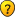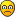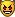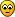## conversion factors

Try to post your problem here, there is a chance, someone clever will help you.
Forum rules
Dear convert-me.com forum visitors,

Our forum has been available for many years. In September 2014 we decided to switch it to read-only mode. Month after month we saw less posts with questions and answers from real people and more spam posts. We were spending more and more resources cleaning the spam until there were less them 1 legitimate message per 100 spam posts. Then we decided it's time to stop.

All the posts in the forum will be available and searchable. We understand there are a lot of useful information and we aren't going to remove anything. As for the new questions, you can always ask them on convert-me.com FaceBook page

Thank you for being with us and sorry for any inconveniences this could caused.

### conversion factors

I am trying to convert 7 liters into grams. How is this possible How can you convert grams into milliliters?
kgreene

### Re: conversion factors

kgreene wrote:I am trying to convert 7 liters into grams. How is this possible How can you convert grams into milliliters?

The original definition of the gram was the mass of 1 cmÂł of water at its maximum density (at 4 Â°C). This idea was carried over into the SI system so that the kilogram is the mass of 1000 cmÂł of water, and this volume is defined as the liter, so that 1 liter of water has a mass of 1 kilogram.
William J. Knight
Health Physicist
Los Alamos National LabsKnight
Expert

Posts: 309
Joined: Tue Jan 13, 2004 9:43 pm
Location: Los Alamos, NM

### Re: Question about fraction

What fraction of a meter is a centimeter? please reply asap.
mbslk32

Posts: 1
Joined: Wed Mar 09, 2005 8:39 am

### Re: conversion factors

Knight wrote:
kgreene wrote:I am trying to convert 7 liters into grams. How is this possible How can you convert grams into milliliters?

The original definition of the gram was the mass of 1 cmÂł of water at its maximum density (at 4 Â°C). This idea was carried over into the SI system so that the kilogram is the mass of 1000 cmÂł of water, and this volume is defined as the liter, so that 1 liter of water has a mass of 1 kilogram.
Guest

### Re: conversion factors

kgreene wrote:I am trying to convert 7 liters into grams. How is this possible How can you convert grams into milliliters?
Guest

### Re: conversion factors

I am trying to convert 22.876 mL into L
dan

### Re: conversion factors

i am trying to convert 1KG of furnace oil into liters and 1KG of LPG (liquified petroleum gas) into liters. Please help.
Ahmed Bukhari

### Re: conversion factors

dan wrote:I am trying to convert 22.876 mL into L

i NEED TO CONVERT 90 GRAMS IN mL
Guest

### Re: conversion factors

Im trying to convert 200 grams of H2SO4 to milliliters. thanks
guest

### Re: conversion factors

Anonymous wrote:
dan wrote:I am trying to convert 22.876 mL into L

i need to convert 2408 grams into kilograms
Guest

### Converting

[/i][/b]
I need to know if 400 milliliters or 4 quarts go into 4 liters equallyIC3~T

### Re: conversion factors

Anonymous wrote:
dan wrote:I am trying to convert 22.876 mL into L

i NEED TO CONVERT 250 grams into millilitres
Guest

### Re: conversion factors

Anonymous wrote:
Anonymous wrote:
dan wrote:I am trying to convert 22.876 mL into L

i NEED TO CONVERT 80 grams into millilitres
Guest

### Re: conversion factors

Anonymous wrote:
dan wrote:I am trying to convert 22.876 mL into L

i need to convert 75mL to L
]
Guest

### Re: conversion factors

Anonymous wrote:
Anonymous wrote:
dan wrote:I am trying to convert 22.876 mL into L

i need to convert 2408 grams into kilograms

22.876 ml divided by 1000mls [which equals 1L]
= 0.022876 L

2408grams divided by 1000grams [which equal 1 kilogram]
= 2kg 408grams.
Guest

### Re: conversion factors

Anonymous wrote:
Anonymous wrote:
dan wrote:I am trying to convert 22.876 mL into L

i NEED TO CONVERT 250 grams into millilitres
Guest

how do u convert leters into grams?
Guest

### Re: conversion factors

i need to convert 4008 grams to miligrams
emily

### help me

i need to convert 500 mL to ______L
???

### Re: conversion factors

"The volume of one can of pop is 355 mL. HOw many L are in one can of pop? HOw many cans of pop could you pour into a 2 L bottle?
Guest

### Re: conversion factors

[quote="kgreene"]I am trying to convert 24 liters into grams. How is this possible How can you convert grams into liters
Guest

### Re: conversion factors

Anonymous wrote:
Anonymous wrote:
Anonymous wrote:
dan wrote:I am trying to convert 22.876 mL into L

i need to convert 2408 grams into kilograms

22.876 ml divided by 1000mls [which equals 1L]
= 0.022876 L

2408grams divided by 1000grams [which equal 1 kilogram]
= 2kg 408grams.

Can someone help me convert these 8:

a) 0.765 g to kilograms
b) 1.34 g to milligrams
c) 34.2 mg to grams
d) 23 745 kg to milliograms (use two conversion factors)

a) 17.3 m to centimeters
b) 2.56 m to kilometers
c) 567 dm to meters
d) 5.13 m to millimeters

HELP WOULD BE GREATLY APPRECIATED!!!
Guest

### Re: conversion factors

[quote="Anonymous]Can someone help me convert these 8:

a) 0.765 g to kilograms
b) 1.34 g to milligrams
c) 34.2 mg to grams
d) 23 745 kg to milliograms (use two conversion factors)

a) 17.3 m to centimeters
b) 2.56 m to kilometers
c) 567 dm to meters
d) 5.13 m to millimeters

HELP WOULD BE GREATLY APPRECIATED!!![/quote]

No, I won't do all your homework for you. You have to:
1) Understand what the prefix means kilo= 1000, milli = 0.001
2) Understand that you can directly change them to/from scientific notation

Doing the first one as an example
0.765 g to kilograms

You want kilograms = 10^3 g
1 = 10^(-3) * 10^3
so 0.765 * 10^(-3) * 10^3 g = 0.765 * 10(-3) kg
= 0.000765 kg.

It is all moving decimals,after a little practice you can do it in your head.
Guest

### Re: conversion factorsAnonymous wrote:
Anonymous wrote:
Anonymous wrote:
dan wrote:I am trying to convert 22.876 mL into L

i need to convert 10.0 ml into L

22.876 ml divided by 1000mls [which equals 1L]
= 0.022876 L

2408grams divided by 1000grams [which equal 1 kilogram]
= 2kg 408grams.dont got one

### how do i.....

i do i convert meters into centimeters?
chocohlic27

Anonymous wrote:how do u convert leters into grams?
Guest

### Re: conversion factors

how do you convert 3g into milligrams?
Guest

### Re: conversion factors

Anonymous wrote:how do you convert 3g into milligrams?

The difference between gram and milligram is just moving the decimal place to the right 3 times so 3g = 3000 mg
Guest

### Re: conversion factors

Anonymous wrote:
kgreene wrote:I am trying to convert 24 liters into grams. How is this possible How can you convert grams into liters
I'm trying to convert liters into kilograms
Guest

### Re: conversion factors

Anonymous wrote:
kgreene wrote:I am trying to convert 24 liters into grams. How is this possible How can you convert grams into liters
Guest

gvb
Guest

### Re: conversion factors

what are these?
46 km=__m
567mg=__g
0.62 kg= __ mg
24.7 g= __mg
329 mL= __ L
0.52 kL= __ mL
how many grams are in 1.01 kilograms?
how many cm are in 6.302 km?
ttyryjfyu

### Re: conversion factors

Anonymous wrote:
Anonymous wrote:
kgreene wrote:I am trying to convert 1 gram to 1 litre. How is this possible How can you convert grams into liters
I'm trying to convert liters into kilograms
Guest

### Re: conversion factors

Anonymous wrote:
Anonymous wrote:
kgreene wrote:I am trying to convert 24 liters into grams. How is this possible How can you convert grams into liters
I'm trying to convert liters into kilograms
Guest

### Re: conversion factors

Anonymous wrote:
Anonymous wrote:
Anonymous wrote:
kgreene wrote:I am trying to convert 1 gram to 1 litre. How is this possible How can you convert grams into liters
I'm trying to convert liters into kilograms
Guest

### Re: conversion factors

Anonymous wrote:
Anonymous wrote:
kgreene wrote:I am trying to convert 2 grams to liters or mililiters. How is this possible How can you convert grams into lmililiters
Guest

### Re: conversion factors

Anonymous wrote:
Anonymous wrote:
kgreene wrote:I am trying to convert 24 liters into grams. How is this possible How can you convert grams into liters
I'm trying to convert liters into kilograms
Guest

### to convert gram into mililliters

[b]sir i m try to convert 25gm into liter but i m not getting it so plz help me to short out this problem[b][size=18][/size][/b]
kaushal

### Re: to convert gram into mililliters

kaushal wrote:sir i m trying to convert 25gm into liter but i m not getting it so plz help me to short out this problem[b]
Guest

### Re: conversion factors

kgreene wrote:I am trying to convert 7 liters into grams. How is this possible How can you convert grams into milliliters?
Guest

### Re: conversion factors

1 miiltre is equals to how many liters
Guest

### Re: conversion factors

i need help!! Im trying to find out how many liters are in 150 cm cubed. I also need to know how many cm are in 100 mL. One more thing... I also need to know how many cm are in 5.0 litersGuest

this crap sucks your never gonna use it why do they even bother teaching it.
Guest

Guest

### Re: conversion factors

kgreene wrote:I am trying to convert 7 liters into grams. How is this possible How can you convert grams into milliliters?
Guest

### Re: conversion factors

Anonymous wrote:
Anonymous wrote:
dan wrote:I am trying to convert 22.876 mL into L

i need to convert 75mL to oz]
Guest

just for everyone
1 liter=100 milliliters
1000 liters=1 kiloliter
100 miligrams=1 gram
1000 grams=1 kilogram
10 millimeters=1 centimeter
100 centimeters=1 meter
1000 meters=1 kilometer
Also 1ml of water is the same as 1 gram or 1cm cubed. Metric measurements are based on this. If you need to convert some other substance to grams from ml or vice versa you need to find the density (mass to volume ratio) and go from there.
Guest

does any one even know? i cant find any replies with the answers
Guest

### Re: conversion factors

Anonymous wrote:
dan wrote:I am trying to convert 22.876 mL into L

i NEED TO CONVERT 300 GRAMS IN mL
Guest

### How do I convert meters cubed to ml

chocohlic27 wrote:i do i convert meters into centimeters?
Guest

### Re: conversion factors

Anonymous wrote:
kgreene wrote:I am trying to convert 7 liters into grams. How is this possible How can you convert grams into milliliters?
Guest

### Re: conversion factors

[How do I convert 175g into ml?
Guest

Anonymous wrote:how do u convert leters into grams?
Guest

### Re: conversion factors

Anonymous wrote:
Anonymous wrote:
dan wrote:I am trying to convert 22.876 mL into L

i NEED TO CONVERT 300 kilometers IN mph
Guest

Anonymous wrote:how do u convert leters into grams?
Guest

### Re: conversion factors

Anonymous wrote:
Anonymous wrote:
kgreene wrote:I am trying to convert dm into L, how do I do that?
Guest

### Re: conversion factors

[how do i convert cm3 into grams?
tinabanina

help
sheila

### TRING TO CONVERT LITERS INTO MILILITERS HELP?I'm tring to help my 9 yrs old grandson convert 8liters into mililiters,Please
HELP.....asap
Thanks
TAE

### Re: TRING TO CONVERT LITERS INTO MILILITERS HELP?

TAE wrote::oops:
I'm tring to help my 9 yrs old grandson convert 8liters into mililiters,Please
HELP.....asap
Thanks,[/list][/code]
Guest

### Re: conversion factors

[quote="Anonymous"][How do I convert 72,760 ml into grams?
Guest

im trying to conver 12566.371cm3 in to liters please help asap
Guest

12566.371 cubic cm = 12.566371 liter

just divide by a thousand, or formally,

liter = cmÂł / 1000 or liter = cmÂł * 0.001
Unit measurement online conversions, calculations and formulas
http://www.aqua-calc.com/
spider
competent

Posts: 74
Joined: Thu Jun 09, 2005 9:57 am
Location: United States

how many leters make a milliliter?
Guest

### Re: conversion factors

Anonymous wrote:
Knight wrote:
kgreene wrote:I am trying to convert 7 liters into grams. How is this possible How can you convert grams into milliliters?

The original definition of the gram was the mass of 1 cmÂł of water at its maximum density (at 4 Â°C). This idea was carried over into the SI system so that the kilogram is the mass of 1000 cmÂł of water, and this volume is defined as the liter, so that 1 liter of water has a mass of 1 kilogram.
Guest

### Re: conversion factors

Anonymous wrote:
Anonymous wrote:
dan wrote:I am trying to convert 25ml into cm3
Guest

Anonymous wrote:just for everyone
1 liter=100 milliliters
1000 liters=1 kiloliter
100 miligrams=1 gram
1000 grams=1 kilogram
10 millimeters=1 centimeter
100 centimeters=1 meter
1000 meters=1 kilometer
Also 1ml of water is the same as 1 gram or 1cm cubed. Metric measurements are based on this. If you need to convert some other substance to grams from ml or vice versa you need to find the density (mass to volume ratio) and go from there.
Guest

### Re: conversion factors

Trying to convert 5.0 L into mL
Guest

### Re: conversion factors

Anonymous wrote:
Knight wrote:
kgreene wrote:I am trying to convert 7 liters into grams. How is this possible How can you convert grams into milliliters?

The original definition of the gram was the mass of 1 cmÂł of water at its maximum density (at 4 Â°C). This idea was carried over into the SI system so that the kilogram is the mass of 1000 cmÂł of water, and this volume is defined as the liter, so that 1 liter of water has a mass of 1 kilogram.
Guest

Anonymous wrote:just for everyone
1 liter=1000 milliliters
1000 liters=1 kiloliter
100 miligrams=1 gram
1000 grams=1 kilogram
10 millimeters=1 centimeter
100 centimeters=1 meter
1000 meters=1 kilometer
Also 1ml of water is the same as 1 gram or 1cm cubed. Metric measurements are based on this. If you need to convert some other substance to grams from ml or vice versa you need to find the density (mass to volume ratio) and go from there.
Guest

### Re: conversion factors

how can I convert cm3 into litres?
[/u]
salamanderp

### Re: conversion factors

I need to convert 2.4 mL into cm3. How?
patmac

### Re: conversion factors

Anonymous wrote:
kgreene wrote:I am trying to convert 7 liters into grams. How is this possible How can you convert grams into milliliters?
Guest

### Re: conversion factors

Anonymous wrote:
Anonymous wrote:
dan wrote:I am trying to convert 22.876 mL into L

i NEED TO CONVERT 1 cm3 millilitres
Guest

### Re: conversion factors

Anonymous wrote:
dan wrote:I am trying to convert 22.876 mL into L

i NEED TO CONVERT 90 GRAMS IN mL
Guest

### how do you convert 1350 grams into mL (militers)???

Knight wrote:
kgreene wrote:I am trying to convert 7 liters into grams. How is this possible How can you convert grams into milliliters?

The original definition of the gram was the mass of 1 cmÂł of water at its maximum density (at 4 Â°C). This idea was carried over into the SI system so that the kilogram is the mass of 1000 cmÂł of water, and this volume is defined as the liter, so that 1 liter of water has a mass of 1 kilogram.
UR Mother's friend

### how do you convert 1350 grams into mL (militers)???

Knight wrote:
kgreene wrote:I am trying to convert 7 liters into grams. How is this possible How can you convert grams into milliliters?

The original definition of the gram was the mass of 1 cmÂł of water at its maximum density (at 4 Â°C). This idea was carried over into the SI system so that the kilogram is the mass of 1000 cmÂł of water, and this volume is defined as the liter, so that 1 liter of water has a mass of 1 kilogram.
UR Mother's friend

### Re: conversion factors

Anonymous wrote:
dan wrote:I am trying to convert 22.876 mL into L

i NEED TO CONVERT 450ml into cubic cm
Guest

### Re: conversion factors

Anonymous wrote:
Knight wrote:
kgreene wrote:I am trying to convert 7 liters into grams. How is this possible How can you convert grams into milliliters?

The original definition of the gram was the mass of 1 cmÂł of water at its maximum density (at 4 Â°C). This idea was carried over into the SI system so that the kilogram is the mass of 1000 cmÂł of water, and this volume is defined as the liter, so that 1 liter of water has a mass of 1 kilogram.
Guest

### Re: conversion factors

Anonymous wrote:
dan wrote:I am trying to convert 22.876 mL into L

i NEED TO CONVERT 40 GRAMS IN ml
Guest

### converting grams to mL

guest wrote:Im trying to convert grams to milliliters. thanks
ashlee

### Re: conversion factors

whats the conversion from ml to grams?
i need to find 50 ml in grams..
Guest

### Re: conversion factors

Anonymous wrote:[quote="Anonymous]Can someone help me convert these 8:

a) 0.765 g to kilograms
b) 1.34 g to milligrams
c) 34.2 mg to grams
d) 23 745 kg to milliograms (use two conversion factors)

a) 17.3 m to centimeters
b) 2.56 m to kilometers
c) 567 dm to meters
d) 5.13 m to millimeters

HELP WOULD BE GREATLY APPRECIATED!!![/quote]

No, I won't do all your homework for you. You have to:
1) Understand what the prefix means kilo= 1000, milli = 0.001
2) Understand that you can directly change them to/from scientific notation

Doing the first one as an example
0.765 g to kilograms

You want kilograms = 10^3 g
1 = 10^(-3) * 10^3
so 0.765 * 10^(-3) * 10^3 g = 0.765 * 10(-3) kg
= 0.000765 kg.

It is all moving decimals,after a little practice you can do it in your head.[/quote]
Guest

### ml to g

How do u convert ml to grams is the liquid u are wanting to convert isn't water. I know 50ml's of my liquid weighs 61g... Then need to convert it to mg/l gahhhh... >< confused!### Re: conversion factors

Anonymous wrote:
dan wrote:I am trying to convert 22.876 mL into L

i NEED TO CONVERT 275mL to L]
me

### Re: conversion factors

how do i convert 720 grams into litres
Guest

### Re: conversion factors

guest wrote:Im trying to convert 0.67655 grams of ethanol
to milliliters. thanks
Guest

### Grams

I'm not sure how to do this but i need to know how to convert grams into liters
Steph

### Re: TRING TO CONVERT LITERS INTO MILILITERS HELP?

just divide by 100, or move the decimal 2 places to the right
kerkery

### Re: conversion factors

me wrote:
Anonymous wrote:
dan wrote:I am trying to convert 22.876 mL into L

i NEED TO CONVERT 10 to the power 6mL to litres
Guest

### Re: conversion factors

me wrote:
Anonymous wrote:
dan wrote:I am trying to convert 22.876 mL into L

i NEED TO CONVERT 275mL to L]

I need to convert 474 liters to kiloliters
Guest

### Re: conversion factors

Trying to convert 474 L to kilo
Guest

l to ml
Guest

### Re: conversion factors

dan wrote:I am trying to convert 22.876 mL into L

Simply divide by 1000

22.876 divided my 1000 = 0.022876 or 2.3 x 10 to the power of 2
Guest

### Re: conversion factors

I am trying to convert 249.4 grams into kg
Guest

### Re: conversion factors

Anonymous wrote:
Anonymous wrote:
dan wrote:I am trying to convert 22.876 mL into L

i NEED TO CONVERT 250 grams into millilitres
Guest

### Re: conversion factors

me wrote:
Anonymous wrote:
dan wrote:I am trying to convert 22.876 mL into L

i NEED TO CONVERT 275mL to L]

there are 1000 ml in 1 L. that's how easy it is
Guest

### Re: conversion factors

I need to convert 1 liters to kiloliters[/quote]
Guest

### Re: conversion factors

ttyryjfyu wrote:what are these?
46 km=__m
567mg=__g
0.62 kg= __ mg
24.7 g= __mg
329 mL= __ L
0.52 kL= __ mL
how many grams are in 1.01 kilograms?
how many cm are in 6.302 km?
Guest

Anonymous wrote:
Anonymous wrote:how do u convert leters into grams?
divide by a thousand
Guest

Next